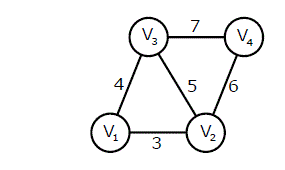Open in App
Not now

# Algorithms | Graph Minimum Spanning Tree | Question 1

• Last Updated : 28 Jun, 2021

An undirected graph G(V, E) contains n ( n > 2 ) nodes named v1 , v2 ,….vn. Two nodes vi , vj are connected if and only if 0 < |i – j| <= 2. Each edge (vi, vj ) is assigned a weight i + j. A sample graph with n = 4 is shown below. What will be the cost of the minimum spanning tree (MST) of such a graph with n nodes? (GATE CS 2011)(A) 1/12(11n^2 – 5n)
(B) n^2 – n + 1
(C) 6n – 11
(D) 2n + 1